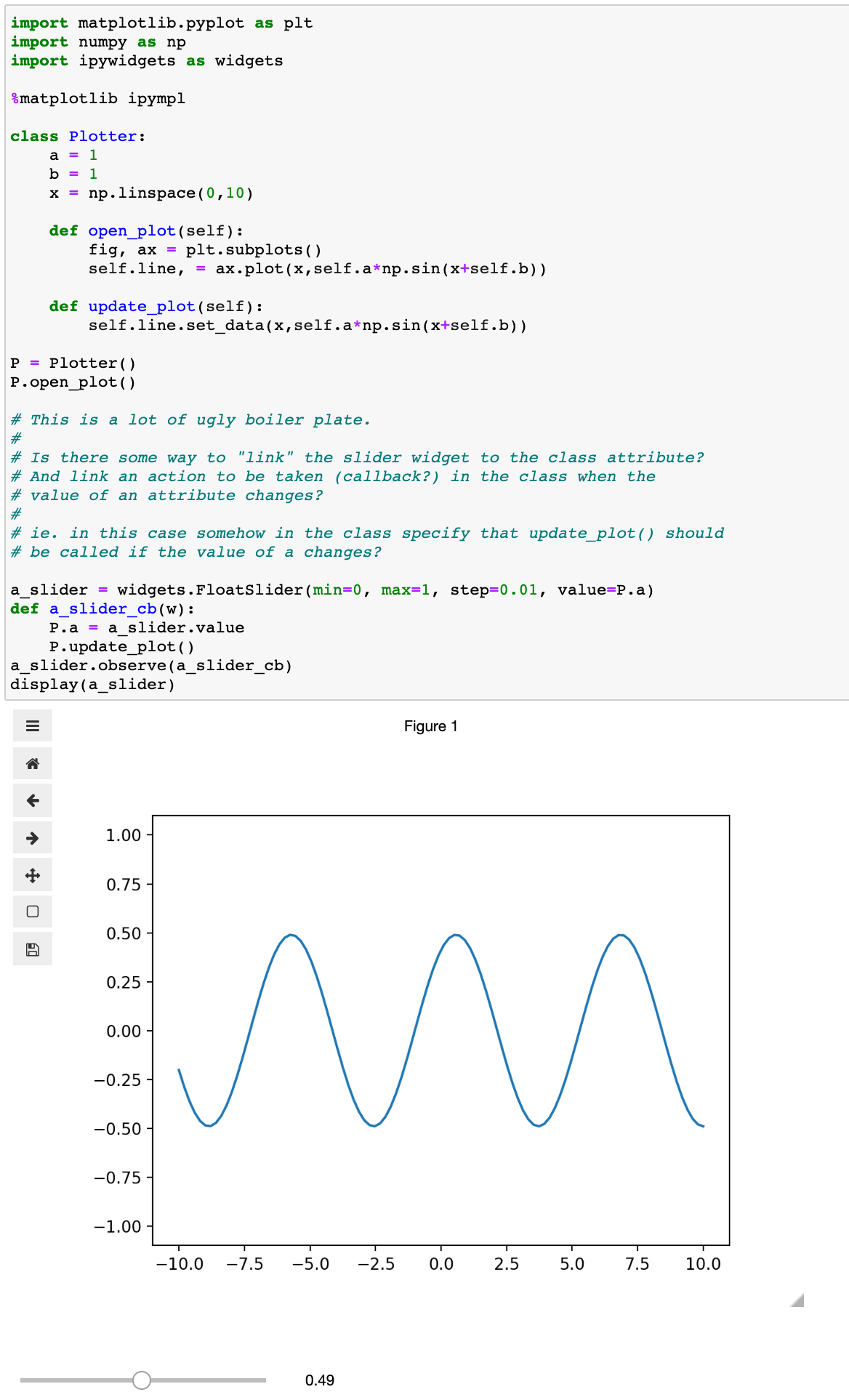# "linking" widgets to class attributes and class attributes to actions

Hi all,

A bit related to my previous question Python question: “observer pattern” actions for dictionary?:

I would like to build an interaction of a class with an ipywidget. Currently, I do this with a painful piece of boilerplate using callbacks (a bad habit from dirty C++ programming with FLTK…). But I feel this can be done in a much better way.

To be concrete, he is a minimalistic example of what I want to do:gary-misc-notebooks/Linking class variables and a widget.ipynb at master · gsteele13/gary-misc-notebooks · GitHub

It works, but the thing I don’t like is the last bit of linking the slider to the class attribute and an action:

``````a_slider = widgets.FloatSlider(min=0, max=1, step=0.01, value=P.a)
def a_slider_cb(w):
P.a = a_slider.value
P.update_plot()
a_slider.observe(a_slider_cb)
``````

It’s ugly. And a pain to code. And in a larger project where I want to have 100+ sliders in tabs interacting with two plots at the same time via a class with hundreds of attributes, I feel I should be looking for a better way.

My question is then: is there an elegant, pythonic way to generate this linking and action?

In particular it seems it would make sense to associate / link the value of the slider widget to the value of the class attribute, when creating the slider. And then the logical place to define the action to be taken would be in the class itself, as it likely know what action should be taken if `a` changes.

@slavoutich mentioned trailets in my previous post. Could these be a way to do this? And if so, could you show how to modify my minimalistic code to use them?

Thanks!
Gary

Indeed, if you make `P` a subclass of `traitlets.HasTraits` with `a` a trait, you’d be able to use `traitlets.link(a_slider, "value", P, "a")`.

2 Likes

Yep, linking with trailets is great!

``````import matplotlib.pyplot as plt
import numpy as np
import ipywidgets as widgets
from traitlets import HasTraits, link, Float, observe

%matplotlib ipympl

# Plotter is in this way a subclass of HasTraits
class Plotter(HasTraits):
a = Float(1.0)
b = Float(0)
x = np.linspace(0,10)

def open_plot(self):
fig, ax = plt.subplots()
self.line, = ax.plot(x,self.a*np.sin(x+self.b))

@observe('a', 'b')
def update_plot(self, value):
self.line.set_data(x,self.a*np.sin(x+self.b))

# Create object, open plot
P = Plotter()
P.open_plot()
``````

Above we subclass HasTraits and decorate our callbacks with a list attributes that should observe them

And then here is the pretty compact code for creating the widgets and linking them, here now for both plot parameters in 4 lines

``````# Trailet linking, this is a lot nicer!
a_slider = widgets.FloatSlider(min=0, max=1, step=0.01, value=P.a)